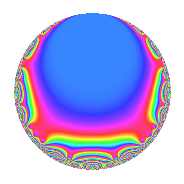# Properties

 Label 189.2.s.aLevel 189 Weight 2 Character orbit 189.s Analytic conductor 1.509 Analytic rank 0 Dimension 2 CM No Inner twists 2

# Learn more about

## Newspace parameters

 Level: $$N$$ = $$189 = 3^{3} \cdot 7$$ Weight: $$k$$ = $$2$$ Character orbit: $$[\chi]$$ = 189.s (of order $$6$$ and degree $$2$$)

## Newform invariants

 Self dual: No Analytic conductor: $$1.5091725982$$ Analytic rank: $$0$$ Dimension: $$2$$ Coefficient field: $$\Q(\sqrt{-3})$$ Coefficient ring: $$\Z[a_1, a_2]$$ Coefficient ring index: $$1$$ Sato-Tate group: $\mathrm{SU}(2)[C_{6}]$

## $q$-expansion

Coefficients of the $$q$$-expansion are expressed in terms of a primitive root of unity $$\zeta_{6}$$. We also show the integral $$q$$-expansion of the trace form.

 $$f(q)$$ $$=$$ $$q$$ $$+ ( 1 + \zeta_{6} ) q^{2}$$ $$+ \zeta_{6} q^{4}$$ $$+ 3 q^{5}$$ $$+ ( -3 + \zeta_{6} ) q^{7}$$ $$+ ( 1 - 2 \zeta_{6} ) q^{8}$$ $$+O(q^{10})$$ $$q$$ $$+ ( 1 + \zeta_{6} ) q^{2}$$ $$+ \zeta_{6} q^{4}$$ $$+ 3 q^{5}$$ $$+ ( -3 + \zeta_{6} ) q^{7}$$ $$+ ( 1 - 2 \zeta_{6} ) q^{8}$$ $$+ ( 3 + 3 \zeta_{6} ) q^{10}$$ $$+ ( -1 + 2 \zeta_{6} ) q^{11}$$ $$+ ( -1 - \zeta_{6} ) q^{13}$$ $$+ ( -4 - \zeta_{6} ) q^{14}$$ $$+ ( 5 - 5 \zeta_{6} ) q^{16}$$ $$+ ( -3 + 3 \zeta_{6} ) q^{17}$$ $$+ ( -6 + 3 \zeta_{6} ) q^{19}$$ $$+ 3 \zeta_{6} q^{20}$$ $$+ ( -3 + 3 \zeta_{6} ) q^{22}$$ $$+ ( 3 - 6 \zeta_{6} ) q^{23}$$ $$+ 4 q^{25}$$ $$-3 \zeta_{6} q^{26}$$ $$+ ( -1 - 2 \zeta_{6} ) q^{28}$$ $$+ ( 6 - 3 \zeta_{6} ) q^{29}$$ $$+ ( -4 + 2 \zeta_{6} ) q^{31}$$ $$+ ( 6 - 3 \zeta_{6} ) q^{32}$$ $$+ ( -6 + 3 \zeta_{6} ) q^{34}$$ $$+ ( -9 + 3 \zeta_{6} ) q^{35}$$ $$-7 \zeta_{6} q^{37}$$ $$-9 q^{38}$$ $$+ ( 3 - 6 \zeta_{6} ) q^{40}$$ $$+ ( -3 + 3 \zeta_{6} ) q^{41}$$ $$-\zeta_{6} q^{43}$$ $$+ ( -2 + \zeta_{6} ) q^{44}$$ $$+ ( 9 - 9 \zeta_{6} ) q^{46}$$ $$+ ( 8 - 5 \zeta_{6} ) q^{49}$$ $$+ ( 4 + 4 \zeta_{6} ) q^{50}$$ $$+ ( 1 - 2 \zeta_{6} ) q^{52}$$ $$+ ( 5 + 5 \zeta_{6} ) q^{53}$$ $$+ ( -3 + 6 \zeta_{6} ) q^{55}$$ $$+ ( -1 + 5 \zeta_{6} ) q^{56}$$ $$+ 9 q^{58}$$ $$+ ( 8 + 8 \zeta_{6} ) q^{61}$$ $$-6 q^{62}$$ $$- q^{64}$$ $$+ ( -3 - 3 \zeta_{6} ) q^{65}$$ $$+ 4 \zeta_{6} q^{67}$$ $$-3 q^{68}$$ $$+ ( -12 - 3 \zeta_{6} ) q^{70}$$ $$+ ( -2 + 4 \zeta_{6} ) q^{71}$$ $$+ ( -3 - 3 \zeta_{6} ) q^{73}$$ $$+ ( 7 - 14 \zeta_{6} ) q^{74}$$ $$+ ( -3 - 3 \zeta_{6} ) q^{76}$$ $$+ ( 1 - 5 \zeta_{6} ) q^{77}$$ $$+ ( -8 + 8 \zeta_{6} ) q^{79}$$ $$+ ( 15 - 15 \zeta_{6} ) q^{80}$$ $$+ ( -6 + 3 \zeta_{6} ) q^{82}$$ $$+ 15 \zeta_{6} q^{83}$$ $$+ ( -9 + 9 \zeta_{6} ) q^{85}$$ $$+ ( 1 - 2 \zeta_{6} ) q^{86}$$ $$+ 3 q^{88}$$ $$-3 \zeta_{6} q^{89}$$ $$+ ( 4 + \zeta_{6} ) q^{91}$$ $$+ ( 6 - 3 \zeta_{6} ) q^{92}$$ $$+ ( -18 + 9 \zeta_{6} ) q^{95}$$ $$+ ( -2 + \zeta_{6} ) q^{97}$$ $$+ ( 13 - 2 \zeta_{6} ) q^{98}$$ $$+O(q^{100})$$ $$\operatorname{Tr}(f)(q)$$ $$=$$ $$2q$$ $$\mathstrut +\mathstrut 3q^{2}$$ $$\mathstrut +\mathstrut q^{4}$$ $$\mathstrut +\mathstrut 6q^{5}$$ $$\mathstrut -\mathstrut 5q^{7}$$ $$\mathstrut +\mathstrut O(q^{10})$$ $$2q$$ $$\mathstrut +\mathstrut 3q^{2}$$ $$\mathstrut +\mathstrut q^{4}$$ $$\mathstrut +\mathstrut 6q^{5}$$ $$\mathstrut -\mathstrut 5q^{7}$$ $$\mathstrut +\mathstrut 9q^{10}$$ $$\mathstrut -\mathstrut 3q^{13}$$ $$\mathstrut -\mathstrut 9q^{14}$$ $$\mathstrut +\mathstrut 5q^{16}$$ $$\mathstrut -\mathstrut 3q^{17}$$ $$\mathstrut -\mathstrut 9q^{19}$$ $$\mathstrut +\mathstrut 3q^{20}$$ $$\mathstrut -\mathstrut 3q^{22}$$ $$\mathstrut +\mathstrut 8q^{25}$$ $$\mathstrut -\mathstrut 3q^{26}$$ $$\mathstrut -\mathstrut 4q^{28}$$ $$\mathstrut +\mathstrut 9q^{29}$$ $$\mathstrut -\mathstrut 6q^{31}$$ $$\mathstrut +\mathstrut 9q^{32}$$ $$\mathstrut -\mathstrut 9q^{34}$$ $$\mathstrut -\mathstrut 15q^{35}$$ $$\mathstrut -\mathstrut 7q^{37}$$ $$\mathstrut -\mathstrut 18q^{38}$$ $$\mathstrut -\mathstrut 3q^{41}$$ $$\mathstrut -\mathstrut q^{43}$$ $$\mathstrut -\mathstrut 3q^{44}$$ $$\mathstrut +\mathstrut 9q^{46}$$ $$\mathstrut +\mathstrut 11q^{49}$$ $$\mathstrut +\mathstrut 12q^{50}$$ $$\mathstrut +\mathstrut 15q^{53}$$ $$\mathstrut +\mathstrut 3q^{56}$$ $$\mathstrut +\mathstrut 18q^{58}$$ $$\mathstrut +\mathstrut 24q^{61}$$ $$\mathstrut -\mathstrut 12q^{62}$$ $$\mathstrut -\mathstrut 2q^{64}$$ $$\mathstrut -\mathstrut 9q^{65}$$ $$\mathstrut +\mathstrut 4q^{67}$$ $$\mathstrut -\mathstrut 6q^{68}$$ $$\mathstrut -\mathstrut 27q^{70}$$ $$\mathstrut -\mathstrut 9q^{73}$$ $$\mathstrut -\mathstrut 9q^{76}$$ $$\mathstrut -\mathstrut 3q^{77}$$ $$\mathstrut -\mathstrut 8q^{79}$$ $$\mathstrut +\mathstrut 15q^{80}$$ $$\mathstrut -\mathstrut 9q^{82}$$ $$\mathstrut +\mathstrut 15q^{83}$$ $$\mathstrut -\mathstrut 9q^{85}$$ $$\mathstrut +\mathstrut 6q^{88}$$ $$\mathstrut -\mathstrut 3q^{89}$$ $$\mathstrut +\mathstrut 9q^{91}$$ $$\mathstrut +\mathstrut 9q^{92}$$ $$\mathstrut -\mathstrut 27q^{95}$$ $$\mathstrut -\mathstrut 3q^{97}$$ $$\mathstrut +\mathstrut 24q^{98}$$ $$\mathstrut +\mathstrut O(q^{100})$$

## Character Values

We give the values of $$\chi$$ on generators for $$\left(\mathbb{Z}/189\mathbb{Z}\right)^\times$$.

 $$n$$ $$29$$ $$136$$ $$\chi(n)$$ $$1 - \zeta_{6}$$ $$\zeta_{6}$$

## Embeddings

For each embedding $$\iota_m$$ of the coefficient field, the values $$\iota_m(a_n)$$ are shown below.

For more information on an embedded modular form you can click on its label.

Label $$\iota_m(\nu)$$ $$a_{2}$$ $$a_{3}$$ $$a_{4}$$ $$a_{5}$$ $$a_{6}$$ $$a_{7}$$ $$a_{8}$$ $$a_{9}$$ $$a_{10}$$
17.1
 0.5 + 0.866025i 0.5 − 0.866025i
1.50000 + 0.866025i 0 0.500000 + 0.866025i 3.00000 0 −2.50000 + 0.866025i 1.73205i 0 4.50000 + 2.59808i
89.1 1.50000 0.866025i 0 0.500000 0.866025i 3.00000 0 −2.50000 0.866025i 1.73205i 0 4.50000 2.59808i
 $$n$$: e.g. 2-40 or 990-1000 Significant digits: Format: Complex embeddings Normalized embeddings Satake parameters Satake angles

## Inner twists

Char. orbit Parity Mult. Self Twist Proved
1.a Even 1 trivial yes
63.s Even 1 no

## Hecke kernels

This newform can be constructed as the kernel of the linear operator $$T_{2}^{2}$$ $$\mathstrut -\mathstrut 3 T_{2}$$ $$\mathstrut +\mathstrut 3$$ acting on $$S_{2}^{\mathrm{new}}(189, [\chi])$$.